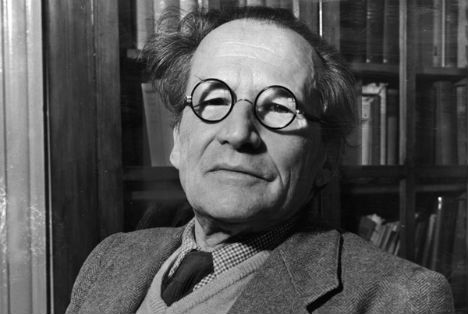# Seven brainteasers to honour Schrödinger

Erwin Rudolf Josef Alexander Schrödinger (1887- 1961) was a prize-winning Austrian physicist. Can you get your head around these brainteasers constructed in honour of his 128th birthday?Photo: akg-images

Schrödinger developed a number of fundamental results in the field of quantum theory, which formed the basis of wave mechanics. He formulated the wave equation (stationary and time-dependent Schrödinger equation) and revealed the identity of his development of the formalism and matrix mechanics. He is also more popularly known for his “Schrödinger's cat” thought-experiment, which asked if a living thing can be alive and dead at the same time.

August 12th, 2015 marks Schrödinger’s 128th birthday. In his honour, electrical engineer Aziz Inan of the University of Portland, Oregon, has constructed the following numerical brainteasers.

1. Schrödinger’s 128th birthday matches his birth date. How so? If his birthday number 128 is interpreted in the day-month calendar date format as 12-8, it corresponds to Schrödinger’s birth date, 12 August. Isn’t this a fun coincidence?

2. Further, number 128 is Schrödinger’s highest-power birthday to occur so far since 128 is 2 to the power of 7. Interestingly enough, if the places of digits 2 and 7 in 2^^7 are switched, 7^^2 yields 49, which equals the sum of the prime factors of 2015 (which are 5, 13, and 31).

3. If numbers 1 to 30 are assigned to the letters of the German alphabet as A being 1, B is 2, C 3, etc., the numbers assigned to the letters of “Erwin” and “Schrödinger” each add up to 69 and 133 respectively and twice the difference of these two numbers yield 128.

4. Also, 128 plus the reverse of 69 (“Erwin”) equals 128 + 96 = 224 and coincidentally, the 224th day of any non-leap year (such as 2015) is Schrödinger’s birth date, August 12th. Wow! In addition, four-thirds of 96 equal 128.

5. Note that 128 = 2^^7, where digits 2 and 7 differ by 5. Interestingly enough, 7 x 2^^5 = 224.

6. The reverse of 128 is 821 where 821 happens to be the 142nd prime number. Now, start from December 31st as being day one and count the calendar dates of a year backwards. Which day corresponds to the 142nd day? (Answer: August 12th!)

7. If Schrödinger’s 128th birthday is written as 12-8-2015 and split into numbers 12, 8, 20, and 15, these numbers add up to 55, which coincides with the sum of the numbers assigned to the letters of Schrödinger’s middle name, “Josef”. Also, January 4th 2016 will mark the 55th anniversary of Schrödinger’s death.

Happy 128th birthday, Erwin Schrödinger!